﻿ New Prospective on Multiple Dice Rolling Game and Its Statistical ImplicationsPublications are Open
Access in this journal
Article Versions
Export Article
• Normal Style
• MLA Style
• APA Style
• Chicago Style
Research Article
Open Access Peer-reviewed

New Prospective on Multiple Dice Rolling Game and Its Statistical Implications

Jimbo Henri Claver , Jawad Azimi, Takeru Suzuki
American Journal of Applied Mathematics and Statistics. 2017, 5(5), 169-174. DOI: 10.12691/ajams-5-5-3
Published online: December 26, 2017

Abstract

We present a mathematical formulation of the Multiple Dice Rolling (MDR) game and develop an adaptive computational algorithm to simulate such game over time. We use an extended version of the well-known Chapman-Kolmogorov Equations (CKEs) to model the state transition of the probability mass function of each side of the dice during the game and represent the time-dependent propensity of the game by a simple regression process, which enable to capture the change in the expectation over time. Furthermore, we perform a quantitative analysis on the outcome of the game in a framework of Average Probability Value (APV) of appearance of a side of the dice over trials. The power of our approach is demonstrated. Our results also suggest that in the MDR game, the APV of appearance of a side of a dice can be appropriately predicted independently of the number of sides and trials.

1. Introduction

We consider a Multiple Dice Rolling (MDR) game in a theoretic-analytic prospective. Hypothetical, the model can be describe by N - sided dices and n trials under the supervision of a third party or referee; such dice may not be a square anymore. As mentioned in 1, 2, 3, MDR game is particularly longstanding and pervasive due to the infinite richness of the game. The quantitative understanding of dice game, both in terms of probability theory and statistical laws, remains difficult and unclear. Investigations are complex due to the shortage of reliable data on the one hand, and the game randomness and unclear interactions on the other hand. Information about outcome of the game is often difficult to quantify and not available in large numbers. While for a single unbiased six-side die rolling game, the probability mass function (pmf) and the statistic of the game could be easily calculated, not much is known about mechanism of formation of these probabilities in the case of multiple dice rolling (MDR) game. With the recent advances in computer science, and the appearance of extensive databases, it is now possible to make dice games with reasonable numbers of dice accessible to quantitative analysis, even for large numbers of throws 4, 5, but this has not yet been done with multiple dice.

As has pointed out in 6, one of the major problems with the MDR game is the difficulty to simultaneously follow the dynamic of all sides of the dice over time. To overcome such a limitation, we assume without loss of generality that “we throw the dice and follow the dynamic somehow separately”. By studying the dynamic of the probability distribution of each side of a die over many throws or trials, we intend to estimate the average Probability Value (APV) of the appearance of each side of the dice at a fixed number trials. After repeated experiments, these values can then be used to reasonably predict the future outcome of the game.

The MDR problem is subject to stochastic perturbations that can produce delays or change on the outcomes experienced by individual dice during trials. These delays are mainly due to the existence of random collisions and/or propulsions of sides in such a dynamical process. Additionally, we assume that the way the stochastic fluctuations propagate to dice is the result of the propensity allocation and parameterization in the model. In such conditions, the noise process will no longer be independent across dice. On this account, the effect of stochastic fluctuations cannot simply be understood to be redundant.

As we study the distribution of dice sides subject to constant stochastic fluctuations and propensity that randomly affect the delays of individual sides, we must not forget about parsimony in designing the model. Our model is presented in detail in the next section of the paper, where we develop the game-theoretic machinery by introducing a modified version of the “dynamic probability” of dice sides over time and examine its statistical properties. The central aim of this work is to formulate the dice game problem in the framework of Markov processes also known as stochastic games 7. Authors in 4, 6, 8 have developed some analogue of game theoretic-analytical techniques to capturing relevant information in random dynamical systems. While many methods could be applied to this task, we chose a probabilistic approach because of its natural way to deal with games and ability to encode arbitrary dependencies between variables 5, 8, 9.

In the past, many authors have dealt with stochasticity in game theory, 7 built the fundament of probability theory and developed the dynamic concept of probability and applications. 10, 11 introduced the formulation of stochastic games and developed a general and comprehensive approach to such games. In 12, 13, the Bellman equation of the problem and the computation of optimal states were formulated. Other authors such as 2, 12 also strongly contributed in developing various game models. In our work, we follow the idea of A.N. Kolmogorov and develop a probabilistic method for the MDR game. We extend the Chapman-Kolmogorov concept of “dynamic probability” by including not only the random state and time variables but also the model parameter update. This will enable our model to adapt to the changing condition of the game 15, 16, 17.

Again, to support the choice of our approach, it is important to mention that, although in many studies, we are generally more concerned with obtaining the entire cumulative distribution of the outcomes of the game view as a stochastic process, here, we are interested in the marginal distribution of each random variable which is precisely a side of the die. While the computation of the marginal probability distribution of a die is useful in evaluating the stochastic model, we believe that information regarding such distribution may not be good enough for predicting individual side of a dice over trials. In this respect, when the game is incomplete, we need to develop an approach, which will evaluate the average probability of appearance of each side of all dice over trials in terms of min–max average value. This information is crucial as we specifically want to predict the outcome of the overall game. In the next section, we will present the model specification.

2. Model Specifications

2.1. Nearly Gaussian Process for the MDR Game

Let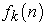be the ‘probability’ function that the k – th side of a die appears after n trials. This function is discrete with continuous analogue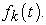We assume such functions as nearly Gaussian process with probability distribution or probability mass function (p.m.f) that a given side k - appears after the n-th trials given as. This analogous of Gaussian distribution is fully characterized by the mean, standard deviation (meanand covariance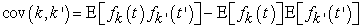whereis the expectation value). Furthermore, we assume that the functions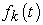are stationary, differentiable and bounded.

A. Chapman-Kolmogorov Equations (CKEs)

The collection of all outcomes of the MDR game, which is an n-dimensional stochastic process can be captured by the mean of Chapman-Kolmogorov Equations (CKEs). In the next section, we further consider a new version of these equations for the dynamic ofover time. Based on the computed probabilities, the game-theoretic model is formulated in terms of the Chapman-Kolmogorov Equations (CKEs), sometimes called the Master equation, where the transition state reflects the change in the outcome after each throw. Until now, such modelling approaches used in many problems do not take into account the random change in the allocation of propensity over time; such a change might be efficiently captured by the change in propensity of the game.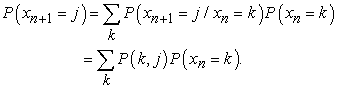(1)

This formulation has some linear algebra interpretation. To go from the probability distribution of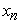to the probability distribution of, we need the adjoint of the matrix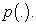B. Extended Chapman-Kolmogorov Equations

In this section, we propose and describe a new version of the Chapman-Kolmogorov Equations. We assume that the drift term also has an independent dynamic, which may be captured with a simple regression model, and that each dynamic is conditioned on the initial state and time. The model is called the Extended Chapman-Kolmogorov Equations (ECKEs) for the MDDR game and is formulated as follows:(2)

Where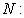Total number of dice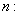Total number of throws (rolling)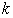: Number of sides of a die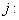j-th Number of throws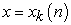: Event “side k appears after n throws”: Probability mass function of side k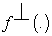: Transpose of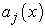: Propensity for side k of the j-th throw;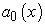: Initial propensity for side k: State change associated with a single event at the j-th throw: Probability that k-th side appears in the intervalat the j-th throw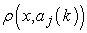: Correlation between events (sides) at the j-th throw and propensity. We assume for convenience that each side of a die is strongly correlated with the parameter of the game for each iteration, and we set the following: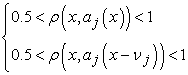(3)

As the choice of the propensity will closely depend on the related event, we assume that the absence of restriction on the correlations will affect the probability value as well, but yet, we cannot confirm whether it will decrease or increase. This will be investigated in our next work. Further, we know that in some situations, the functioncould be too hard to explicitly obtain. Hence, we propose an approximation ofassuming that the random variable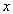has a negative exponential distribution:and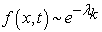for every t, where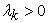is the parameter of the distribution. Additionally, without loss of generality, we apply some consideration of the Fréchet derivative (refer to the subsections 2.2. and 2.3. below). We can, without loss of generality, approximate the first derivative of f (.) toand the equation (2) becomes:(4)

After simplification, this system of equations becomes(5)

From the above equations, we compute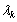as a function of the side number k and the iteration period j; that is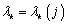. We have that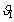and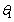are the constant parameters of the game, which are assumed to be known. In our particular case, we set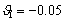andfor convenience. Also we assume that the initial propensity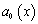is known andis a vector with a finite number of elements which represents the delay of all sides. This setup leads us to the following algorithm.

2.2. Definition

Let V and W be Banach spaces and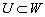be an open subset of V. A function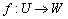is called Frechet differentiable at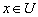if there exists a bounded operator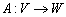such that.

2.3. Consequence

The consequence of having the limit of a function defined on metric spaces (U and W) is that, there exists a sequence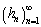of non-zero element V, which converges to zero vector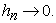Thus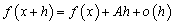andis called Frechet derivative of f at x.

3. Our Algorithm

As the analytical solution to (1) is hard to obtain, even for a moderate number of throws, a numerical algorithm using an adapted stochastic simulation approach on equation (4) is proposed in this paper. In our algorithm, two random variables determine the temporal evolution of the game. The variableis the time for the next event to occur (next appearance a side of dice) and k, as before, is the side of the dice. Further, the probability density of an event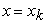is then evaluated based on the propensity of the game on the event involved.

Overall, this will give a better flexibility and applicability of the algorithm in some sense. The main purpose of creating such an algorithm is to simultaneously simulate the game and predict the online probability mass functions of each event using model (1). One important remark here is that the probability mass function f at each throw is a vector, because we throw many dice at once and each side has a certain probability of appearance. We shall use f transpose instead of f to ensure better readability of our outputs, but all properties remain the same. Finally, our algorithm facilitates the evaluation of the Average Probability Value (APV) of each side after a certain number of throws; this is important if we want to study the general trend of the game too. Our algorithm is presented as follows:

Start

Input: Initial data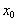Outputs:1. Set.

2. For t = 1: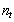(= number of iterations) do

a. Let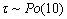be the time until the next event, which changes over time.

b. Let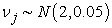be the change associated to a single event.

c. Compute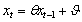and.

d. Compute the value of the pmfbased upon eq (4).

e. Compute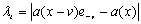.

3. Output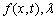End

In the next section, we will show some limitations of the MDR game.

B. Limitations

The main concern with the Multiple Dice Rolling game is the lack of robust test data in which the dynamic interactions are known in advance. We need the data in which the true correlations are known if we want to compare our approach with the existing methodology. It is not possible to find the exact values for these probabilities, first because the source of noise is not unique and also because of the noise error measurements in the system is not easy to obtain. One way to relax this requirement is to introduce additional parameters, which will influence the computation of probabilities. Afterward build a new generation of flexible algorithms, which create test data sets and accurately predict outcomes of the game over time.

Here, we proposed a propensity allocation based approach in order for our model to generate and predict the state of the game, but other researchers may prefer more complex function. Finally, we present the test datasets created by our technique, which can be used to assess the performance of algorithms that attempt to determine the underlying predicted state. Our algorithm is available for download, and the test datasets are also available in additional files and may be used to repeat the experiment if desired.

3.1. Test Dataset

The main motivation for creating a Test Dataset was to have an initial dataset for which we may presume to know certain regulatory rules. This dataset will be necessary to compare outcomes of games with various other datasets and the accuracy being used. This is because until now there is no existent data for multiple dice rolling games for which the true underlying interaction and propensity allocation are exactly known. We, therefore, create a sample data set by permuting in a random manner the numbers 1 to 6 as many times as we need.

We anticipate that the availability of the test dataset will allow researchers to evaluate their own methods and compare their methods against commonly used algorithms. While the test data we provide will be useful for researchers who want to get started right away testing their algorithms, we emphasize that the real power of the proposed algorithm is the capability it provides to quickly produce necessary outcomes for a game when a good allocation of propensity is made. Further, interested researchers can also use their own test datasets to compare the dependency of any method on any particular parameter (number of sides of a dice, type of correlation among sides, type of data, type of propensity etc…) in an efficient manner. In the section that follows, we will present the normal unbiased game.

3.2. Normal “Unbiased” Dice Game

We throw 1000 six – sides dice each, 1000 times. Each side will randomly appear each time with some probability.

• Figure 1. Sample paths of the probability values for all dice sides with the permutation Dataset. We assign a color to each side; the blue color represents side 1, green side 2, red side 3, grey side 4, violet side 5 and finally, yellow side 6
• Figure 2. Histogram of each side of die in MDR game. The sample paths of probability values and the pmf of each side at each throw

• View option

• View option

4. Characterization of MRD Game

A. Robustness

The proposed model will not be robust against a game that is biased. If we assume that the roller can influence the throw of the dice or use any other trick to influence the outcome, such games will not be robust. But, if we consider that the rolling conditions are the same and the dice are identical all the time, then the game becomes robust.

B. Simplicity

The model of MDR game is extremely simple and straightforward. Additional constraints might slightly affect some properties of the game and/or even change the APV(K) of the MDR game over trials.

C. Efficiency

The model efficiency will depend on the initial conditions of the game, the propensity, the number of iterations and the number of dice rolled and the model parameters. When the number of dice increase infinitely, the game becomes more difficult to analyze.

5. Conclusion and Discussion

In this paper, a model of MRD game using both mathematical tools and computational algorithm in order to estimate the average probability of appearance of each side of a dice over trials is presented and analyzed. The proposed algorithm makes it possible to detect interactions among all dice sides as dynamic correlations and evaluates the p.m.f of all sides to appear over throws. We use the variance-covariance matrix to extract necessary information in the game over time.

The input of our algorithm is associated to a matrix where columns represent the dice thrown and rows represent the side of dice. The outcome of games in terms of the prediction or p.m.f values greatly depends on the propensity and the parameters of our model. We found that the time complexity of the proposed algorithm was polynomial of orderwhere n is the number throws.

We have shown how a very simple mathematical model can be used to capture the stochasticity in the MDR game. The extended version of the Chapman-Kolmogorov Equations used in this paper is still a simplification, and could even be unrealistic for certain designs of the game, --but it can capture the randomness of the game if we use good initial conditions and reasonably stable parameters. The main novelty of our approach is the introduction of the propensity of the game and the application of our algorithm to predict the average probability of appearance of each side of a die over throws. However in practice, when using MDR to solve real world problems, it is not enough to find the average probability value. Again, all outcomes in our model depend on the initial inputs and the parameters. We then have to continuously update new probability values as dice are thrown.

We face the challenge of iteratively improving the solution to a series of problems that change over time, and we must do this in light of unanticipated events with incomplete knowledge. The fact that uncertainty and inaccuracy enter into our model, the prediction must be accepted and even used to the great possible benefit.

Acknowledgements

We are grateful to all colleagues for discussions and suggestions, which helped us to improve the presentation of this paper. The work presented in this paper was supported by the SAKURA Research Grant and AUAF Research and Development Fund. We thank Hamidullah Hamidy for helping in reviewing this work. This publication reflects only the author’ views and any remaining mistakes are ours.

References

  Ferguson, T. S. (1968). Mathematical statistics - A decision theoretic approach. Academic Press, New York. In article View Article  Krasovskij, N. N. (1970), Game theoretic problem of capture. Nauka, Moscow. In article  Murray, H. J. R. (2002). A history of chess. Oxford University Press, London, Reprint. In article  Alon, N. & Krilievich, M. (2006). Extremal and probabilistic combinatory. Canad. J. Math, 11:34-38. In article  Backwell, D. & Girshick, M.A. (1954) Theory of games and statistical decisions. John Wiley & Sons, New York. In article View Article  Conway, J. H. (1976). On numbers and games. Academic Press, New York. In article View Article  Kolmogorov, A.N. (1988). Foundation of the theory of probability. (3rd eds). Phasis, Moscow. In article PubMed  Aumann, R.J. (1989). Lecture note on Game Theory, Westview Press Inc., Boulder, Colorado In article  Iverson, G. R. & Longcour, W. H. (1971). Bias and runs in dice throwing and recording: A Few million throws. Psychometrika, 36, 01. In article View Article  Kreps, D. M. (1990) Game theory and Economic Modeling, Oxford University Press. In article View Article  PubMed  Maitra, A. P. & Sudderth, W. D. (1996). Discrete gambling and stochastic games: Applications of mathematics 32. Springer-Verlag. In article View Article  Morris, P. (1994) Introduction to game theory. Springer-Verlag, New York. In article View Article  Roth, A. E. & Sotomayor, M. A. O. (1990). Two sides matching- A study in game theoretic modeling and analysis. Cambridge University Press. In article View Article  Feller, W. (1972). An introduction to probability theory and its applications. John Wiley & Son, New York, vol. 2. In article View Article  Jimbo, H.C. (2004). Distribution characterization in a practical moment problem. Acta Mathematica, no 4, 73, 1. In article View Article  Kac. J. L, Logan, L. L. (1976). Fluctuating phenomena. Eds. E.S.W. Montroll & J:L: Lebowitz, North Holland, Amsterdam. In article  Knifer R. (1999). Dice game properly explained. Elliot Right Wy Books. ISBN 0-7160-2112-9W. In articleThis work is licensed under a Creative Commons Attribution 4.0 International License. To view a copy of this license, visit http://creativecommons.org/licenses/by/4.0/